### Reports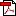266 kb
Date : 26 Jun 2014
Annex-2 : Methodologies

Macroeconomic Stability Map

The Macroeconomic Stability Map is based on six sub-indices, each pertaining to a specific area of macroeconomic risk. Each sub-index on macroeconomic risk includes select parameters representing risks in that particular field. These sub-indices were selected based on their impact on macroeconomic or financial variable such as GDP, inflation, interest rates or the assets quality of banks. A per cent rank over the sample period is used to standardise each ratio. The standardised ratios are combined using weights to calculate the index for each dimension. The six sub-indices of the overall macroeconomic stability index and their components are briefly described here.

Global Index: The global index is based on the output growth of the world economy. A fall in output growth affects overall sentiments for the domestic economy in general and has implications on demand for domestic exports in particular. Capital flows to the domestic economy are also affected by growth at the global level. Therefore, a fall in output growth is associated with increased risks.

Domestic Growth Index: The domestic growth index is based on growth of gross domestic product. A fall in growth, usually creates headwinds for banks’ asset quality, capital flows and overall macroeconomic stability. Hence, a fall in growth is associated with increased risks.

Inflation Index: Inflation based on the Consumer Price Index (combined) is used to arrive at the inflation index. Increase in inflation reduces the purchasing power of individuals and complicates investment decision of corporates. Therefore, an increase in inflation is associated with higher risks.

External Vulnerability Index: The current account deficit (CAD) to GDP ratio, reserve cover of imports and ratio of short-term external debt to total external debt are included in the external vulnerability index. Rising CAD and the ratio of short-term external debt to total external debt and a falling reserve cover of imports depict rising vulnerability.

Fiscal Index: The fiscal index is based on fiscal and primary deficit. Higher deficits are associated with higher risks. High government deficit, in general, reduces the resources available to the private sector for investment and also has implications for inflation.

Corporate Index: The health of the corporate sector is captured through the profit margin (earnings before interest, tax, depreciation and amortisation [EBITDA] to sales) and the interest coverage ratio (earnings before interest, tax [EBIT] to interest payments). A lower profit margin and lower interest coverage ratio are associated with higher risks.

Corporate Sector Stability and Map

The Corporate sector Stability Map have been constructed using the following method:

Data: The balance sheet data of non-government non-financial public limited companies.

Frequency: Annual (1992-93 to 2013-14). For 2012-13 and 2013-14, the half-yearly financial statements of listed non-government non-financial companies have been used for the analysis.

Following ratios have been used for the analysis (considering 5 dimensions):

a. Profitability : RoA(Gross Profit/Total Assets) #, Operating Profit/Sales #, Profit After Tax/Sales #;

b. Leverage : Debt/ Assets, Debt/ Equity; (Debt is taken as Total Borrowings)

c. Sustainability : Interest Coverage Ratio (EBIT to interest expenses) #, interest expenses/total expenditure;

d. Liquidity : Quick Assets/ Current Liabilities (quick ratio) #;

e. Turn-Over : Total Sales / Total Assets #.

Note: # Negatively related to risk.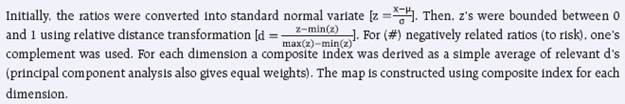Banking Stability Map and Indicator

The Banking Stability Map and Indicator (BSI) presents an overall assessment of changes in underlying conditions and risk factors that have a bearing on the stability of the banking sector during a period. The following ratios are used for constructing each composite index:

 Table 1: Ratios used for constructing the Banking Stability Map and Banking Stability Indicator Dimension Ratios Soundness CRAR # Tier-I Capital to Tier-II Capital # Leverage ratio as Total-Assets to Capital and Reserves Asset-Quality Net NPAs to Total-Advances Gross NPAs to Total-Advances Sub-Standard-advances to gross NPAs # Restructured-Standard-Advances to Standard-Advances Profitability Return on Assets # Net Interest Margin # Growth in Profit # Liquidity Liquid-Assets to Total-Assets # Customer-Deposits to Total-Assets # Non-Bank-Advances to Customer-Deposits Deposits maturing within-1-year to Total Deposits Efficiency Cost to Income Business (Credit + Deposits) to staff expenses # Staff Expenses to Total Expenses Note: # Negatively related to risk.

The five composite indices represent the five dimensions of soundness, asset-quality, profitability, liquidity and efficiency. Each composite index, representing a dimension of bank functioning, takes values between zero (minimum) and 1 (maximum). Each index is a relative measure during the sample period used for its construction, where a high value means the risk in that dimension is high. Therefore, an increase in the value of the index in any particular dimension indicates an increase in risk in that dimension for that period as compared to other periods. For each ratio used for a dimension, a weighted average for the banking sector is derived, where the weights are the ratio of individual bank assets to total banking system assets. Each index is normalised for the sample period as ‘ratio-on-a-given-date minus minimum-value-in-sample-period divided by maximum-valuein- sample-period minus minimum-value-in-sample-period’. A composite index of each dimension is calculated as a weighted average of normalised ratios used for that dimension, where the weights are based on the marks assigned for assessment for the CAMELS rating. Based on the individual composite index for each dimension, the Banking Stability Indicator is constructed as a simple average of these five composite sub-indices.

Banking Stability Measures (BSMs) – Distress Dependency Analysis

In order to model distress dependency, the methodology described by Goodhart and Segoviano (2009) was followed. First, the banking system has been conceptualised as a portfolio of banks (BIs). Then, the PoD of individual banks, comprising the portfolio, has been inferred from equity prices. Subsequently, using such PoDs as inputs (exogenous variables) and employing the Consistent Information Multivariate Density Optimizing (CIMDO) methodology (Segoviano 2006), which is a non-parametric approach based on cross-entropy, the banking system’s portfolio multivariate density (BSMD) was derived. Lastly, from BSMD a set of conditional PoDs of specific pairs of BIs, and the banking system’s joint PoD (JPoD) are estimated.

BSMD and thus the estimated conditional probabilities and JPoD, embed the banks’ distress dependency. This captures the linear (correlation) and non-linear dependencies among the BIs in the portfolio, and allow for these to change throughout the economic cycle. These are key advantages over traditional risk models that most of the time incorporate only correlations, and assume that they are constant throughout the economic cycle.

Estimation of Losses: Expected Loss, Unexpected Loss and Expected Shortfall of SCBs

The following standard definitions were used for estimating these losses:

 Expected Loss (EL) : EL is the average credit loss that the banking system expects from its credit exposure. Unexpected Loss (UL) : Unexpected Loss (UL): UL at 100(1-α) per cent level of significance is the loss that may occur at the α-quantile of the loss distribution. Expected Shortfall (ES) : Expected Shortfall (ES): When the distributions of loss (Z) are continuous, expected shortfall at the 100(1-α) per cent confidence level (ESα (Z)) is defined as, ESα (Z) = E[Zα Z≥VaRα (Z)]. Hence, Expected Shortfall is the conditional expectation of loss given that the loss is beyond the VaR level.

These losses were estimated as: Loss = PD X LGD X EAD

Where, EAD = Exposure at Default, is the total advances of the banking system. EAD includes only on-balance sheet items as PD was derived only for on-balance sheet exposures.

LGD = Loss Given Default. Under the baseline scenario, the average LGD was taken as 60 per cent as per the RBI guidelines on ‘Capital Adequacy – The IRB Approach to Calculate Capital Requirement for Credit Risk’. LGD was taken at 65 per cent and 70 per cent under medium and severe macroeconomic conditions respectively.

PD = Probability of Default. PD was defined as gross non-performing advances to total advances ratio. Because of unavailability of data on a number of default accounts, the size of default accounts (that is, the NPA amount) was used for derivation of PDs.

The above losses, EL, UL and ES, were estimated by using a simulated PD distribution. As a first step an empirical distribution of the PD was estimated using the Kernel Density Estimate; second using the empirically estimated probability density function, 20,000 random numbers were drawn based on the Monte Carlo simulation and finally, EL, UL and ES were calculated, by taking PDs as average PD, 99.9 per cent VaR of PD and average PD beyond 99.9 per cent loss region respectively.

Macro Stress Testing

To ascertain the resilience of banks against macroeconomic shocks, a macro stress test for credit risk was conducted. Here, the credit risk indicator was modelled as a function of macroeconomic variables, using various econometric models that relate the banking system aggregate to macroeconomic variables. The time series econometric models used are: (i) multivariate regression in terms of the slippage ratio; (ii) aggregate VAR using slippage ratio; (iii) quantile regression of slippage ratio; (iv) multivariate panel regression on bank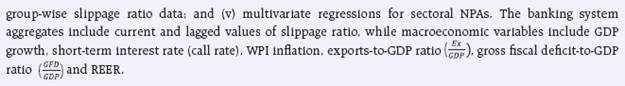While multivariate regression allows evaluating the impact of selected macroeconomic variables on the banking system’s NPA and capital, the VAR model reflects the impact of the overall economic stress situation on the banks’ capital and NPA ratio, which also take into account the feedback effect. In these methods, conditional mean of slippage1 ratio is estimated and it is assumed that the impact of macro-variables on credit quality will remain the same irrespective of the level of the credit quality, which may not always be true. In order to relax this assumption, quantile regression was adapted to project credit quality, in which in place of conditional mean the conditional quantile was estimated.

The Modelling Framework

The following multivariate models were run to estimate the impact of macroeconomic shocks on the GNPA ratio/ slippage ratio (SR):2

System Level Models

The projection of system level GNPAs are done using three different but complementary econometric models: multivariate regression, vector autoregressive (which takes into account the feedback impact of credit quality to macro variables and interaction effects) and quantile regression (which can deal with tail risks and takes into account the non-linear impact of macroeconomic shocks). The average of projections derived from these models is used for calculation of impact on CRAR.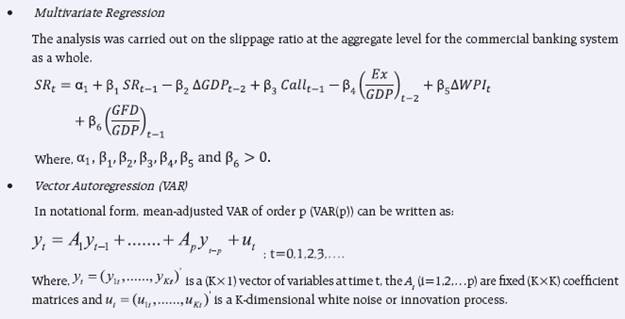In order to estimate, the VAR system, slippage ratio, call rate, inflation, growth and REER were selected. The appropriate order of VAR was selected based on minimum information criteria as well as other diagnostics and suitable order was found to be 2. Accordingly, VAR of order 2 (VAR(2)) was estimated and the stability of the model was checked based on roots of AR characteristic polynomial. Since all roots are found to be inside the unit circle, this selected model was found fulfil the stability condition. The impact of various macroeconomic shocks was determined using the impulse response function of the selected VAR.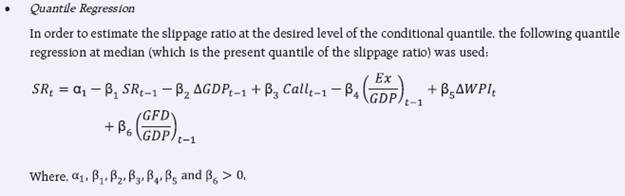Bank Group Level Models

The projection of bank groups-wise GNPA are done using three different but complementary econometric models: panel regression, vector autoregressive (which takes into account the feedback impact of credit quality to macro-variables and interaction effects) and quantile regression (which can deal with tail risks and takes into account the non-linear impact of macroeconomic shocks). The average of projections derived from these models is used for calculation of impact on CRAR.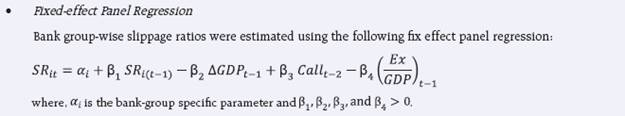• Vector Autoregression

In order to model the slippage ratio of various bank groups, different VAR models of order 2 were estimated based on the following macro variables:

PSBs: Real GDP growth, WPI-inflation, call money rate and REER.
OPBs: Real GDP growth, call money rate and exports to GDP ratio.
NPBs: Real GDP growth, WPI-inflation, call money rate and exports to GDP ratio.
FBs: Real GDP growth, WPI-inflation, call money rate and exports to GDP ratio.

• Quantile Regression

In order to model the slippage ratio of various bank groups, the following quantile regressions for different bank groups were used: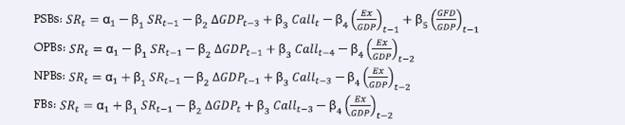Sector Level Models

• Sectoral multivariate regression

The impact of macroeconomic shocks on various sectors was assessed by employing multivariate regression models using aggregate NPA ratio for each sector separately. The dependent variables consisted of lagged NPAs, sectoral GDP growth, infl ation and short-term interest rates.

Derivation of the NPAs from the slippage ratios, which were projected from the earlier mentioned credit risk econometric models, were based on the following assumptions: credit growth of 15 per cent; recovery rate of 6.8 per cent, 5.7 per cent, 5.7 per cent and 5.4 per cent during March, June, September and December quarters respectively; write-offs rate of 5.8 per cent, 3 per cent, 2.3 per cent and 4 per cent during March, June, September and December respectively.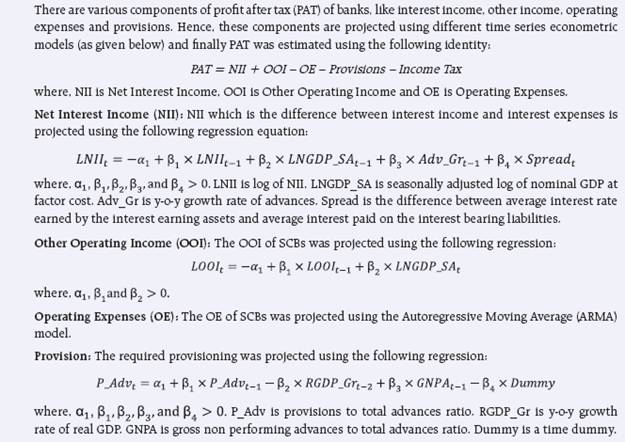Income Tax: The required income tax was taken as 32 per cent of the profit before tax, which is based on the past trend of ratio of income tax to profit before tax.

Finally, impact on CRAR was estimated based on the PAT estimated as mentioned earlier. RWA growth was assumed at 17.7 per cent under the baseline, 20.6 per cent under medium risk and 23.5 per cent under severe risk scenarios. The regulatory capital growth is assumed to remain at the minimum by assuming minimum mandated transfer of 25 per cent of the profit to the reserves account. The projected values of the ratio of the non-performing advances were translated into capital ratios using the ‘balance sheet approach’, by which capital in the balance sheet is affected via provisions and net profits.

Single Factor Sensitivity Analysis – Stress Testing

As a part of quarterly surveillance, stress tests are conducted covering credit risk, interest rate risk, liquidity risk etc. Resilience of commercial banks in response to these shocks is studied. The analysis is done on individual scheduled commercial banks as well as on the aggregated-system.

Credit Risk

To ascertain the resilience of banks, the credit portfolio was given a shock by increasing NPA levels for the entire portfolio. For testing the credit concentration risk, default of the top individual borrower(s) and the largest group borrower was assumed. The analysis was carried out both at the aggregate level as well as at the individual bank level, based on supervisory data as on 31 March 2014. The assumed increase in NPAs was distributed across sub-standard, doubtful and loss categories in the same proportion as prevailing in the existing stock of NPAs. The provisioning norms used for these stress tests were based on existing average prescribed provisioning for different asset categories. The provisioning requirements were taken as 25, 75 and 100 per cent for sub-standard, doubtful and loss advances respectively. These norms were applied on the additional NPAs, calculated under a stress scenario. As a result of assumed increase in NPAs, loss of income on the additional NPAs for one quarter was also included in total losses in addition to additional provisioning requirements. The estimated provisioning requirements so derived were deduced from banks’ capital and stressed capital adequacy ratios were derived.

Interest Rate Risk

Under assumed shocks of the shifting of the INR yield curve, there could be losses on account of the fall in value of the portfolio or decline in income These estimated losses were reduced from the banks’ capital to arrive at stressed CRAR.

For interest rate risk in the trading portfolio (HFT + AFS), a duration analysis approach was considered for computing the valuation impact (portfolio losses). The portfolio losses on these investments were calculated for each time bucket based on the applied shocks. The resultant losses/gains were used to derive the impacted CRAR. In a separate exercise for interest rate shocks in the HTM portfolio, valuation losses were calculated for each time bucket on interest bearing assets using the duration approach. The valuation impact for the tests on the HTM portfolio was calculated under the assumption that the HTM portfolio would be marked to market.

For the interest rate risk impact from the earning perspective on the banking book, the income approach was considered (income losses). Income losses on interest bearing exposure gap, are calculated for one year only for each time bucket separately to reflect the impact on the current year profit and loss and income statements.

Liquidity Risk

The aim of the liquidity stress tests is to assess the ability of a bank to withstand unexpected liquidity drain without taking recourse to any outside liquidity support. The analysis was done as at end-March 2014. Various scenarios depict different proportions (depending on the type of deposits) of unexpected deposit withdrawals on account of sudden loss of depositors’ confidence and assess the adequacy of liquid assets available to fund them.

Assumptions in the liquidity stress tests include:

• It is assumed that banks would meet stressed withdrawal of deposits through sale of liquid assets only.

• The sale of investments is done with a haircut of 10 per cent of their market value.

• The stress test is done on a static mode.

Bottom-up Stress Testing

Bottom-up sensitivity analysis was performed by 22 scheduled commercial banks (comprising about 70 per cent of the total assets). A set of common scenarios and shock sizes were provided to select banks. The tests were conducted using March 2014 data. Banks used their own methodologies for calculating losses in each case.

Stress Testing of the Derivatives Portfolios of Select Banks

The stress testing exercise focused on the derivatives portfolios of a representative sample set of top 24 banks in terms of notional value of the derivatives portfolios. Each bank in the sample was asked to assess the impact of stress conditions on their respective derivatives portfolios.

In case of domestic banks, the derivatives portfolio of both domestic and overseas operations was included. In case of foreign banks, only the domestic (Indian) position was considered for the exercise. For derivatives trade where hedge effectiveness was established it was exempted from the stress tests, while all other trades were included.

The stress scenarios incorporated four sensitivity tests consisting of the spot USD/INR rate and domestic interest rates as parameters

 Table 2: Shocks for Sensitivity Analysis Shock 1 Domestic Interest Rates Overnight +2.5 percentage points Upto 1yr +1.5 percentage points Above 1yr +1.0 percentage points Shock 2 Domestic Interest Rates Overnight -2.5 percentage points Upto 1yr -1.5 percentage points Above 1yr -1.0 percentage points Shock 3 Exchange rates USD/INR +20 per cent Shock 4 Exchange Rates USD/INR -20 per cent

Scheduled Urban Co-operative Banks

Credit Risk

Stress tests on credit risk were conducted on SUCBs using their asset portfolios as at end March 2014. The tests were based on a single factor sensitivity analysis. The impact on CRAR was studied under four different scenarios. The assumed scenarios were:

• Scenario I: 50 per cent increase in GNPA (classified into sub-standard advances).

• Scenario II: 50 per cent increase in GNPA (classified into loss advances).

• Scenario III: 100 per cent increase in GNPA (classified into sub-standard advances).

• Scenario IV: 100 per cent increase in GNPA (classified into loss advances).

Liquidity Risk

Liquidity stress test based on cash flow basis in 1-28 days time bucket was also conducted, where mismatch (negative gap [cash inflow less than cash outflow]) exceeding 20 per cent of outflow was considered stressful.

• Scenario I: Cash outflows in 1-28 days time bucket goes up by 50 per cent (no change in cash inflows).

• Scenario II: Cash outflows in 1-28 days time bucket goes up by 100 per cent (no change in cash inflows).

Non-Banking Financial Companies

Credit Risk

Stress tests on credit risk were conducted on non-banking financial companies (including both deposit taking and non-deposit taking and systemically important) using their asset portfolio as at end-March 2014. The tests were based on a single factor sensitivity analysis. The impact on CRAR was studied under two different scenarios:

• Scenario I: GNPA increased 2 times from the current level.

• Scenario II: GNPA increased 5 times from the current level.

The assumed increase in NPAs was distributed across sub-standard, doubtful and loss categories in the same proportion as prevailing in the existing stock of NPAs. The additional provisioning requirement was adjusted from the current capital position. The stress test was conducted at individual NBFCs levels as well as at an aggregate level.

Interconnectedness – Network Analysis

Matrix algebra is at the core of the network analysis, which is essentially an analysis of bilateral exposures between entities in the financial sector. Each institution’s lendings and borrowings with all others in the system are plotted in a square matrix and are then mapped in a network graph. The network model uses various statistical measures to gauge the level of interconnectedness in the system. Some of the most important ones are:

Connectivity: This is a statistic that measures the extent of links between the nodes relative to all possible links in a complete graph.

Cluster Coefficient: Clustering in networks measures how interconnected each node is. Specifically, there should be an increased probability that two of a node’s neighbours (banks’ counterparties in case of the financial network) are also neighbours themselves. A high clustering coefficient for the network corresponds with high local interconnectedness prevailing in the system.

Shortest Path Length: This gives the average number of directed links between a node and each of the other nodes in the network. Those nodes with the shortest path can be identified as hubs in the system.

In-betweeness Centrality: This statistic reports how the shortest path lengths pass through a particular node.

Eigenvector Measure of Centrality: Eigenvector centrality is a measure of the importance of a node (bank) in a network. It describes how connected a node’s neighbours are and attempts to capture more than just the number of out degrees or direct ‘neighbours’ that a node has. The algorithm assigns relative centrality scores to all nodes in the network and a bank’s centrality score is proportional to the sum of the centrality scores of all nodes to which it is connected. In general, for an NxN matrix there will be N different eigen values, for which an eigenvector solution exists. Each bank has a unique eigen value, which indicates its importance in the system. This measure is used in the network analysis to establish the systemic importance of a bank and by far it is the most crucial indicator.

Tiered Network Structures: Typically, financial networks tend to exhibit a tiered structure. A tiered structure is one where different institutions have different degrees or levels of connectivity with others in the network. In the present analysis, the most connected banks (based on their eigenvector measure of centrality) are in the innermost core. Banks are then placed in the mid-core, outer core and the periphery (the respective concentric circles around the centre in the diagrams), based on their level of relative connectivity. The range of connectivity of the banks is defined as a ratio of each bank’s in degree and out degree divided by that of the most connected bank. Banks that are ranked in the top 10 percentile of this ratio constitute the inner core. This is followed by a mid-core of banks ranked between 90 and 70 percentile and a 3rd tier of banks ranked between the 40 and 70 percentile. Banks with connectivity ratio of less than 40 per cent are categorized as the periphery.

Solvency Contagion analysis

The contagion analysis is basically a stress test where the gross loss to the banking system owing to a domino effect of one or more banks failing is ascertained. We follow the round by round or sequential algorithm for simulating contagion that is now well known from Furfine (2003). Starting with a trigger bank i that fails at time 0, we denote the set of banks that go into distress at each round or iteration by Dq, q= 1,2, …For this analysis, a bank is considered to be in distress when its core CRAR goes below 6 per cent. The net receivables have been considered as loss for the receiving bank.

Liquidity Contagion analysis

While the solvency contagion analysis assesses potential loss to the system owing to failure of a net borrower, liquidity contagion estimates potential loss to the system due to the failure of a net lender. The analysis is conducted on gross exposures between banks. The exposures include fund based and derivatives ones. The basic assumption for the analysis is that a bank will initially dip into its liquidity reserves or buffers to tide over a liquidity stress caused by the failure of a large net lender. The items considered under liquidity reserves are: (a) excess CRR balance; (b) excess SLR balance; (c) available marginal standing facility; and (d) available export credit refinance. If a bank is able to meet the stress with the liquidity buffers alone, then there is no further contagion.

However, if the liquidity buffers alone are not sufficient, then a bank will call in all loans that are ‘callable’, resulting in a contagion. For the analysis only short-term assets like money lent in the call market and other very short-term loans are taken as callable. Following this, a bank may survive or may be liquidated. In this case there might be instances where a bank may survive by calling in loans, but in turn might propagate a further contagion causing other banks to come under duress. The second assumption used is that when a bank is liquidated, the funds lent by the bank are called in on a gross basis, whereas when a bank calls in a short-term loan without being liquidated, the loan is called in on a net basis (on the assumption that the counterparty is likely to first reduce its short-term lending against the same counterparty).

1 Slippages are fresh accretion to NPAs during a period. Slippage Ratio = Fresh NPAs / Standard Advances at the beginning of the period.
2 Slippage ratio, exports/GDP, and the call rate are seasonally adjusted.

Top